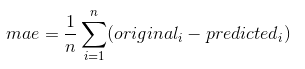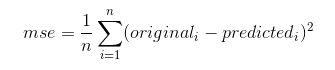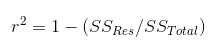# Model Evaluation Metrics in Regression Models with Python

In this tutorial, we are going to see some evaluation metrics used for evaluating Regression models. Whenever a Machine Learning model is being constructed it should be evaluated such that the efficiency of the model is determined, It helps us to find a good model for our prediction by evaluating the model. In such a note, we are going to see some Evaluation metrics for Regression models like Logistic, Linear regression, and SVC  regression.

### Evaluation metrics – Introduction

Generally, we use a common term called the accuracy to evaluate our model which compares the output predicted by the machine and the original data available. Consider the below formula for accuracy,

Accuracy=(Total no. of correct predictions /Total no. of data used for testing)*100

This gives the rough idea of evaluation metrics but it is not the correct strategy to evaluate the model. We have some defined metrics especially for Regression models which we will see below.

### Regression Models Evaluation metrics

The SkLearn package in python provides various models and important tools for machine learning model development. Where it provides some regression model evaluation metrics in the form of functions that are callable from the sklearn package.

• Max_error
• Mean Absolute Error
• Mean Squared Error
• Median Squared Error
• R Squared

Above are the available metrics provided from sklearn we will see them in detail with implementation,

1. Max_error
It calculates the maximum error present between the original data and predicted data,
Where it compares and finds out data that has the maximum difference and produces the output. Consider the code segment below which illustrates the max_error function from the

```from sklearn.metrics import max_error
original_data = [8, 4, 7, 1]
predicted_data = [4, 2, 7, 1]
max_error(original_data,predicted_data)```
```Output:
4```

From the above code, the original data is compared with predicted data, where the maximum difference occurred between data 8 and 4 so the output is the difference between them (i.e  4).
The best output possible here is 0.

2. Mean Absolute Error
It is given by the formula below,Where the difference between data is taken and the average of it is found out and returned as output. The implementation of it is shown in the below code segment.

```from sklearn.metrics import mean_absolute_error
original_data = [3, 5, 2, 7]
predicted_data = [2, 0, 2, 8]
mean_absolute_error(y_true, y_pred)```
```Output:
1.75```

Let us do some calculations here, the difference between these data is 1,5,0,1 (i.e  1+5+0+1) which gives you 7. Then the average is taken where n=4, so 7/4 gives you (1.75).
The best score here would be 0.

3. Mean Squared Error
It is as similar to the above metric wherein Mean Squared Error we will be calculating the square of the difference between the predicted and the original data. The formula is given below,The difference value is calculated and it is squared and means is obtained as the result. Let us see an implementation of it,

```from sklearn.metrics import mean_squared_error
original_data = [3, 5, 2, 7]
predicted_data = [2, 0, 2, 8]
mean_squared_error(original_data,predicted_data)```

The same inputs similar to above mean absolute error is given to this mean squared error, where the difference in the data is ( 1 square+5 square+0 square+1 square) = 27 and mean is (27/4) which gives the output.

```Output:
6.75```

The ideal output is 0 and this suits to identify a very large error in the prediction compared to the mean absolute error.

4. Median Absolute Error
This finds the median value of the absolute difference between the original and the predicted data. It is famous for its consistency towards robust to outliers. It helps us to know about the outliers present in the dataset.

```from sklearn.metrics import median_absolute_error
original_data = [3, 5, 2, 7]
predicted_data = [3, 1, 2, 5]
median_absolute_error(original_data,predicted_data)```
```Output:
1.0```

Let formulate it! , the output of the above code segment is the median(0,4,0,2) that is obviously 1. The best value is 0.

5. R Squared
This is the most important evaluation metric in the regression evaluation where it gives us an understanding of how well the data get fit towards the regression line. This helps us to find the relationship between the independent variable towards the dependent variable.

```from sklearn.metrics import r2_score
original_data = [8, 5, 1, 6]
predicted_data= [7, 8, 2, 3]
r2_score(original_data,predicted_data)```
```Output:
0.23076923076923073```

It is calculated by the below formula,where the SSRes is the sum of the square of the difference between the actual value and the predicted value.SSTotal is the sum of the square of the difference between the actual value and the mean of the actual value.

These are various Regression evaluation metrics available, Hope this tutorial helps!!!

### One response to “Model Evaluation Metrics in Regression Models with Python”

1.Naveen Kumar says:

Super It Was Very Useful .Right blog I Was Searching For…..!